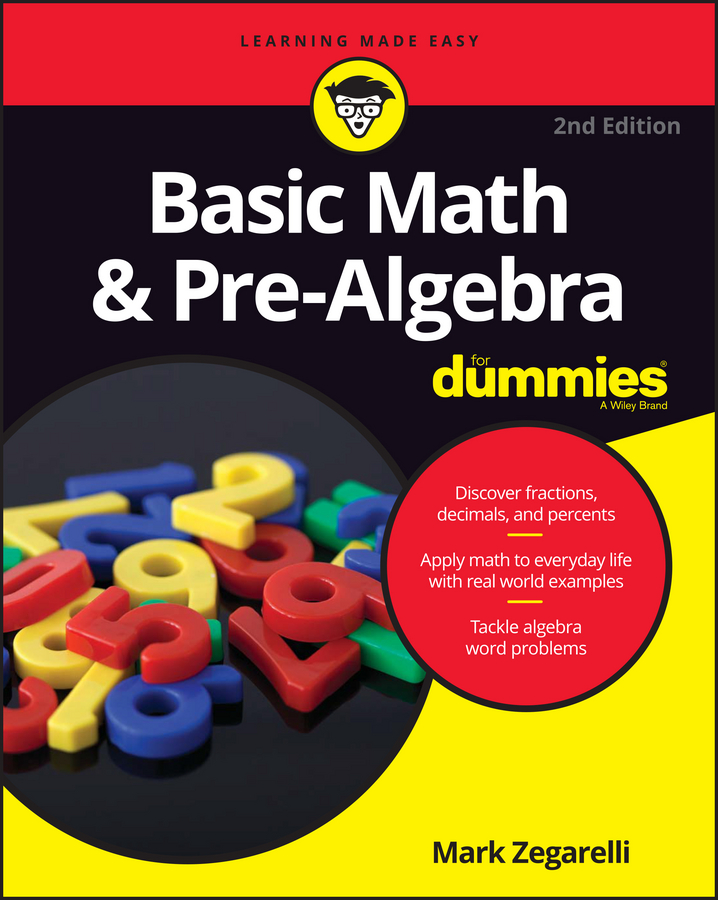##### Basic Math & Pre-Algebra For DummiesWhen an expression has parentheses and powers, evaluate it in the following order: contents of parentheses, powers from left to right, multiplication and division from left to right, and addition and subtraction from left to right.
1. Contents of parentheses

An expression in an exponent (a small, raised number indicating a power) groups that expression like parentheses do. Evaluate any superscripted expression down to a single number before evaluating the power. In other words, to find 53–1, you can pretend 3 – 1 is in parentheses, making the problem 5(3–1) = 52 = 25.

A few other symbols that you may be familiar with also group expressions together just like parentheses. These include the square root symbol and absolute value bars.

2. Powers from left to right

3. Multiplication and division from left to right

4. Addition and subtraction from left to right

## Sample questions

1. Evaluate (8 + 62) / (23 – 4).

11. Begin by evaluating the contents of the first set of parentheses. Inside this set, evaluate the power first and do the addition next:

(8 + 62) / (23 – 4)

= (8 + 36) / (23 – 4)

= 44 / (23 – 4)

Move to the next set of parentheses, evaluating the power first and then the subtraction:

= 44 / (8 – 4) = 44 / 4

Finish up by evaluating the division: 44 / 4 = 11.

2. Find the value of –1 + (–20 + 33)2.

48. When the entire contents of a set of parentheses is raised to a power, evaluate what’s inside the parentheses before evaluating the power. Inside this set, evaluate the power first and the addition next:

–1 + (–20 + 33)2 = –1 + (–20 + 27)2 = –1 + 72

Next, evaluate the power 72 = 7 x 7 = 49:

= –1 + 49

Finish up by evaluating the addition: –1 + 49 = 48

## Practice questions

1. Find (62 – 12) / (16 / 23).

2. Evaluate –10 – (2 + 32 x –4).

3. 72 – (3 + 32 / –9)5 = ?

4. What is (10 – 114 x 8)4/4+5

Following are the answers to the practice questions:

1. (62 – 12) / (16 / 23) = 12.

Focusing on the contents of the first set of parentheses, evaluate the power and then the subtraction:

(62 – 12) / (16 / 23)

= (36 – 12) / (16 / 23)

= 24 / (16 / 23)

Next, work inside the second set of parentheses, evaluating the power first and then the division:

= 24 / (16 / 8) = 24 / 2

Finish by evaluating the division:

= 24 / 2 = 12

2. –10 – (2 + 32 x –4) = 24.

Focusing on the contents of the parentheses, evaluate the power first, then the multiplication, and then the addition:

–10 – (2 + 32 x –4) = –10 – (2 + 9 x – 4) = –10 – (2 + –36) = –10 – (–34)

Finish by evaluating the subtraction:

–10 – (–34) = 24

3. 72 – (3 + 32 / –9)5 = 17.

Focusing inside the parentheses, evaluate the power first, then the division, and then the addition:

72 – (3 + 32 / –9)5

=72 – (3 + 9 / –9)5

=72 – (3 + –1)5

=72 – 25

Next, evaluate both powers in order:

= 49 – 25 = 49 – 32

To finish, evaluate the subtraction:

49 – 32 = 17

4. (10 – 114 x 8)4/4+5 = 64.

Focusing inside the first set of parentheses, evaluate the power first, then the multiplication, and then the subtraction:

(10 – 114 x 8)4/4+5 = (10 – 1 x 8)4/4+5 = (10 – 8)4/4+5 = 24/4+5

Next, handle the expression in the exponent, evaluating the division first and then the addition:

21+5 = 26

To finish, evaluate the power:

26 = 64